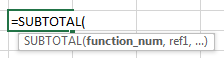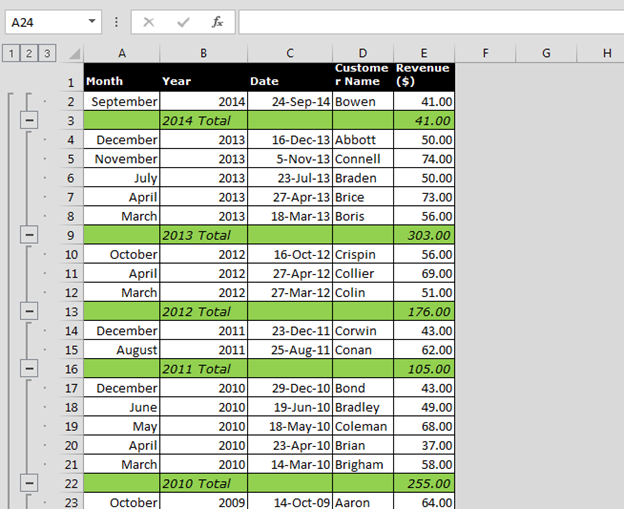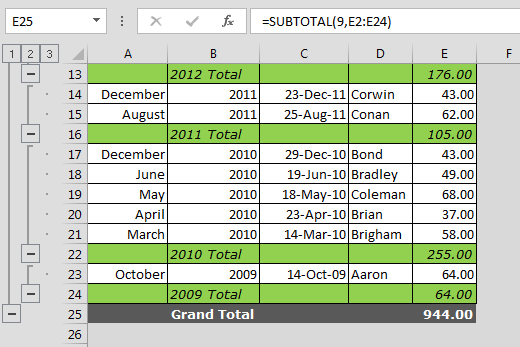Summing Subtotals Only from Range

In this article, we are going to learn how to calculate the sum of the subtotal values only in Microsoft Excel.

Subtotal function is used to return a subtotal in a list or database.Let’s take an example and understand:-

We have revenue data month, year and date wise. Also, we have subtotal for every year. Column B contains six groups of numbers (cells B2, cells B4:8, B10:B12, B14:B15, B17:B21, B23.
Cells B3, B9, B13, B16, B22, and B24 contain SUBTOTAL formulas, which total the numbers to each group.

How can we find the sum of the subtotals values only?• Enter the formula in cell E25.
• =SUBTOTAL(9,E2:E24)
• Press Enter on keyboard.
• Only subtotal number will be calculated.Formula Explanation: -In this formula, subtotal function will help to return the calculation of subtotal numbers.In this formula in subtotal formula, we have used 9 to calculate the sum of subtotal and then select the reference range of subtotal.

This is the way we can use subtotalfunction in Microsoft Excel for different calculations for the subtotal.We would love to hear from you, do let us know how we can improve, complement or innovate our work and make it better for you. Write us at info@exceltip.com

1.2.